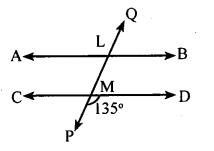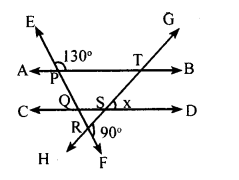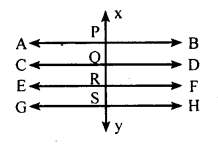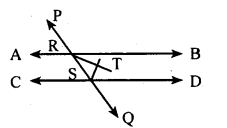# KSEEB Solutions for Class 8 Maths Chapter 3 Axioms, Postulates and Theorems Ex 3.3

In this chapter, we provide KSEEB SSLC Class 8 Maths Chapter 3 Axioms, Postulates and Theorems Ex 3.3 for English medium students, Which will very helpful for every student in their exams. Students can download the latest KSEEB SSLC Class 8 Maths Chapter 3 Axioms, Postulates and Theorems Ex 3.3 pdf, free KSEEB SSLC Class 8 Maths Chapter 3 Axioms, Postulates and Theorems Ex 3.3 pdf download. Now you will get step by step solution to each question.

## Karnataka Board Class 8 Maths Chapter 3 Axioms, Postulates and Theorems Ex 3.3

Question 1.
Find all the angles in the following∠CMP + ∠PMD = 180° [Linear pair]
∠CMP+ 135° = 180°
∠CMP = 180° – 135
∠CMP = 45°
∠LMD = ∠CMP = 45° [Vertical opposite angles]
∠LMC =∠PMD = 135° [Vertical opposite angles]
∠ALM = ∠ LMC = 135° [Alternate angles]
∠BLM = ∠ LMC = 135° [Alternate angle]
∠QLB = ∠LMD = 45° [Corresponding angles]
∠QLA = ∠ LMC = 135° [Corresponding angles

Question 2.
Find the value of x in the diagram below.∠PQS = ∠EPT = 130° [Corresponding angle]
∠PQS + ∠SQR = 180° [Linear pair]
SQR = 180 – 130
∠SQR = 50°
∠QRS + ∠FRS = 180° [Linear pair]
∠QRS + 90° =180°
∠QRS = 180 – 90
∠QRS = 90°
∠SQR+ ∠QRS +∠QSR = 180°
[Sum of the angles of triangle is 180° ]
50 + 90 + ∠QSR = 180°
140 + ∠QSR = 180°
∠QSR = 180 – 140
∠QSR =40°
∠TSD = ∠QSR [Vertically opposite angles]
x = 40°.

Question 3.
Show that if a straight line is perpendicular to one of the two or more parallel lines, then it is also perpendicular to the remaining lines.AB−→−∥CD−→−∥EF−→∥GH−→−⋅XY¯¯¯¯¯¯¯¯ interrectsthese lines at P, Q, R and S and XY−→−⊥AB¯¯¯¯¯¯¯
Toprove: XY¯¯¯¯¯¯¯¯⊥CD−→−,XY¯¯¯¯¯¯¯¯⊥GH−→−
Proof :∠XPB = 90° (data)
∠XPB = ∠ PQD = ∠QRF = ∠RSH = 90°

Question 4.
Let A→B and Cb−→ be two parallel lines and PQ−→− be a transversal. Show that the angle bisectors of a pair of two internal angles on the same side of the transversal are perpendicular to each other.Let the bisectors of ∠BRS and∠RSD intersect at T
To prove: RT⊥ST i.e., ∠RTS = 90°
Proof: ∠BRS +∠RSD = 180°
12 ∠BRS + 12 ∠RSD = 12 × 180°
12 ∠BRS = ∠TRS =∠TRB
12 ∠RSQ = ∠TSR =∠TSD
In triangle TRS,
∠TRS +∠TSR + ∠RTS = 180°
90 + ∠RTS = 180°
∠RTS = 90°
∴ RT ⊥ ST

All Chapter KSEEB Solutions For Class 8 maths

—————————————————————————–

All Subject KSEEB Solutions For Class 9

*************************************************

I think you got complete solutions for this chapter. If You have any queries regarding this chapter, please comment on the below section our subject teacher will answer you. We tried our best to give complete solutions so you got good marks in your exam.

If these solutions have helped you, you can also share kseebsolutionsfor.com to your friends.

Best of Luck!!